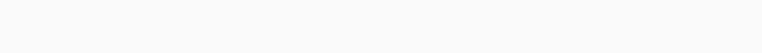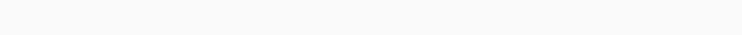## Introduction to SDP

Now we will learn about SDP which is semidefinite programming which was known as the most exciting development in mathematical programming in the 90’s. In this section we will know about what it actually does. Here we go.

As a subfield of convex optimization, semidefinite programming (SDP) involves optimizing a linear objective function over an affine space intersecting with a cone of positive semidefinite matrices (a function that the user wants to minimize or maximize).

In the last ten years, semidefinite programming has been one of the most exciting developments in mathematical programming. The SDP has applications in a variety of fields, including convex constrained optimization, control theory, and combinatorial optimization. Most of these applications can be solved fairly efficiently in practice and in theory by applying interior-point methods to SDP (which usually require the same amount of computing resources as linear optimization).

If an affine combination of symmetric matrices is positive semidefinite, semidefinite programming minimizes a linear function. Positive definite programs are convex optimization problems because the constraint is nonlinear and nonsmooth. Many engineering problems can be solved using semidefinite programming (e.g., linear and quadratic programming). In spite of the fact that semidefinite programs are much more general than linear programs, they are just as easy to solve. Semidefinite programs have been generalized to most interior-point methods for linear programming. The worst-case complexity of these methods is polynomial, and they perform very well in practice. It provides an overview of semidefinite programs and an introduction to primal-dual interior-point methods that can be used to solve them.

Semidefinite programming is a relatively new field of optimization which is of growing interest for several reasons. Many practiPositive definite programs are convex optimization problems. Since such constraints are nonlinear and nonsmooth, they are convex optimization problems.ming problems. In automatic control theory, SDPs are used in the context of linear matrix inequalities. Interior point methods can efficiently solve SDPs, which are in fact a special case of cone programming. Polynomial optimization problems can be approximated using hierarchies of SDPs for linear and (convex) quadratic programs. Complex systems have been optimized using semidefinite programming. Semidefinite programs have been used to formulate some quantum query complexity problems in recent years.

## Application

A semidefinite programming approach has been used to find approximate solutions to combinatorial optimization problems, such as the max cut problem with an approximation ratio of 0.87856. Also, SDPs are used in geometry to determine tensegrity graphs, as LMIs in control theory, and as semidefiniteness constraints in inverse elliptic coefficient problems.

## SDP in Convex Optimization

In convex optimization, SDP has a wide range of applications. Among the types of constraints that can be modeled in the SDP framework are linear inequalities, convex quadratic inequalities, lower bounds on matrix norms, lower bounds on SPSD matrix determinants, lower bounds on nonnegative vector geometric mean, and others. These and other constructions allow you to solve the following problems in the form of semidefinite programs (among many others): linear programming, optimizing convex quadratic forms under convex quadratic inequality constraints, minimizing the volume of an ellipsoid covering a given set of points and ellipsoids, maximizing the volume of an ellipsoid contained within a given polytope, and a variety of maximum and minimum eigenvalue problems. Following are some subsections that show how some convex optimization problems can be reformulated as instances of SDP.

## Let's code

				
%use s2


				
println("Construct the primal and dual SDP problems")

// the primal SDP matrices
val C: SymmetricMatrix = SymmetricMatrix(
arrayOf(
doubleArrayOf(1.0),
doubleArrayOf(2.0, 9.0),
doubleArrayOf(3.0, 0.0, 7.0)
)
)

val A1: SymmetricMatrix = SymmetricMatrix(
arrayOf(
doubleArrayOf(1.0),
doubleArrayOf(0.0, 3.0),
doubleArrayOf(1.0, 7.0, 5.0)
)
)

val A2: SymmetricMatrix = SymmetricMatrix(
arrayOf(
doubleArrayOf(0.0),
doubleArrayOf(2.0, 6.0),
doubleArrayOf(8.0, 0.0, 4.0)
)
)

// construct the primal SDP problem
val primal = SDPPrimalProblem(
C,
arrayOf(A1, A2))

println(primal)// the dual SDP vector and matrices
val b: Vector = DenseVector(11.0, 19.0)
// construct the primal SDP problem
val dual = SDPDualProblem(
b,
C,
arrayOf(A1, A2))

println(dual)/**
* p.465 in
* Andreas Antoniou, Wu-Sheng Lu
*/

println("Solving an SDP problem")

// define an SDP problem with matrices and vectors
val A0: SymmetricMatrix = SymmetricMatrix(
arrayOf(
doubleArrayOf(2.0),
doubleArrayOf(-0.5, 2.0),
doubleArrayOf(-0.6, 0.4, 3.0)
)
)

val A1: SymmetricMatrix = SymmetricMatrix(
arrayOf(
doubleArrayOf(0.0),
doubleArrayOf(1.0, 0.0),
doubleArrayOf(0.0, 0.0, 0.0)
)
)

val A2: SymmetricMatrix = SymmetricMatrix(
arrayOf(
doubleArrayOf(0.0),
doubleArrayOf(0.0, 0.0),
doubleArrayOf(1.0, 0.0, 0.0)
)
)

val A3: SymmetricMatrix = SymmetricMatrix(
arrayOf(
doubleArrayOf(0.0),
doubleArrayOf(0.0, 0.0),
doubleArrayOf(0.0, 1.0, 0.0)
)
)

val A4: SymmetricMatrix = A3.ONE()
val C: SymmetricMatrix = A0.scaled(-1.0)
val b: Vector = DenseVector(0.0, 0.0, 0.0, 1.0)
// construct an SDP problem
val problem = SDPDualProblem(
b,
C,
arrayOf(A1, A2, A3, A4))

// the initial feasible point
val X0: DenseMatrix = DenseMatrix(
arrayOf(
doubleArrayOf(1.0 / 3.0, 0.0, 0.0),
doubleArrayOf(0.0, 1.0 / 3.0, 0.0),
doubleArrayOf(0.0, 0.0, 1.0 / 3.0)
)
)

val y0: Vector = DenseVector(0.2, 0.2, 0.2, -4.0)

val S0: DenseMatrix = DenseMatrix(
arrayOf(
doubleArrayOf(2.0, 0.3, 0.4),
doubleArrayOf(0.3, 2.0, -0.6),
doubleArrayOf(0.4, -0.6, 1.0)
)
)

// the initial central path
val path0: CentralPath = CentralPath(X0, y0, S0)

// solving SDP problem
val solver = PrimalDualPathFollowingMinimizer(
0.9, // γ
0.001) // ε
val solution: IterativeSolution = solver.solve(problem)
val path: CentralPath = solution.search(path0)

//the solution from the textbook is accurate up to epsilon
//changing epsilon will change the answers
// primal solution
println("X = ")
println(path.X)
// dual solution
println("Y = ")
println(path.y)
println("S = ")
println(path.S)/**
* example 15.1 in
* Andreas Antoniou, Wu-Sheng Lu
*/

println("Solving an SQP problem with only equality constraints")

// objective function
val f: RealScalarFunction = object : RealScalarFunction {

override fun evaluate(x: Vector): Double {
val x1: Double = x.get(1)
val x2: Double = x.get(2)
val x3: Double = x.get(3)

var fx: Double = -x1.pow(4.0)
fx -= 2.0 * x2.pow(4.0)
fx -= x3.pow(4.0)
fx -= (x1 * x2).pow(2.0)
fx -= (x1 * x3).pow(2.0)

return fx
}

override fun dimensionOfDomain(): Int {
return 3
}

override fun dimensionOfRange(): Int {
return 1
}
}

// equality constraints
val equality_constraints: EqualityConstraints = GeneralEqualityConstraints(
object : RealScalarFunction {

override fun evaluate(x: Vector): Double {
val x1: Double = x.get(1)
val x2: Double = x.get(2)
val x3: Double = x.get(3)

var fx: Double = x1.pow(4.0)
fx += x2.pow(4.0)
fx += x3.pow(4.0)
fx -= 25.0

return fx // a1
}

override fun dimensionOfDomain(): Int {
return 3
}

override fun dimensionOfRange(): Int {
return 1
}
},
object : RealScalarFunction {

override fun evaluate(x: Vector): Double {
val x1: Double = x.get(1)
val x2: Double = x.get(2)
val x3: Double = x.get(3)

var fx: Double = 8.0 * x1.pow(2.0)
fx += 14.0 * x2.pow(2.0)
fx += 7.0 * x3.pow(2.0)
fx -= 56.0

return fx // a2
}

override fun dimensionOfDomain(): Int {
return 3
}

override fun dimensionOfRange(): Int {
return 1
}
})

// construct an SQP solver
val solver = SQPActiveSetOnlyEqualityConstraint1Minimizer(
object : SQPActiveSetOnlyEqualityConstraint1Minimizer.VariationFactory {
override fun newVariation(
f: RealScalarFunction?,
equal: EqualityConstraints?
): SQPASEVariation {
val impl = SQPASEVariation2(100.0, 0.01, 10)
impl.set(f, equal)
return impl
}
},
1e-8, // epsilon, threshold
20) // max number of iterations
// solving an SQP problem
val solution: IterativeSolution
= solver.solve(f, equality_constraints)
val x: Vector = solution.search(
DenseVector(3.0, 1.5, 3.0), // x0
DenseVector(-1.0, -1.0)) // λ0
val fx: Double = f.evaluate(x)
// print out the solution
println("x = " + x)
println("fx = " + fx)That’s It for this topic. See you soon.